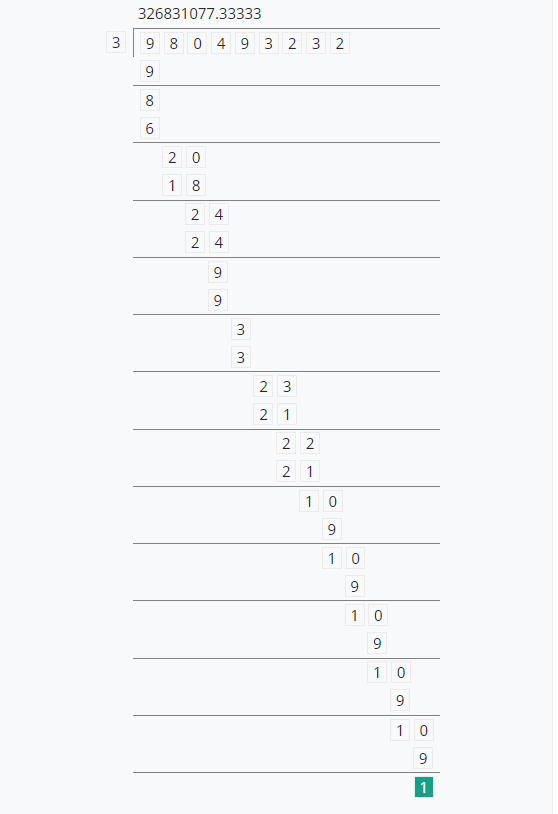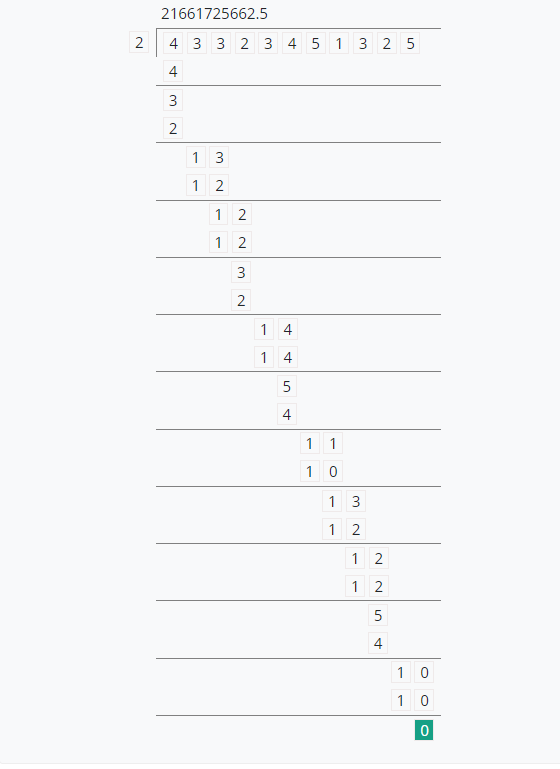# Long Division Calculator with decimals

To find the quotient with decimal, enter the dividend and divisor, and hit the calculate button using long division calculator with decimal

 divisor dividend

Give Us Feedback

# Long Division with Decimal Calculator

Long division with a decimal calculator is used to find the quotient of numbers in decimals.

## What is a long division?

Long division is a process of division and dividing again and again by divisor until the remainder should be zero or a repetition of digits is started in the quotient.

## How to find the quotient in decimals?

Example 1:

Find the quotient when the divisor is 3 and the dividend is 980493232.

Solution

Step 1:

Extract the given data:

Divisor = 3

Dividend = 980493232

Step 2:

Simplifying the problemExample 2:

Find the quotient and remainder when the divisor is 2 and the dividend is 43323451325.

Solution

Step 1:

Extract the given data:

Divisor = 2

Dividend = 43323451325

Step 2:

Simplifying the problem### Math Tools